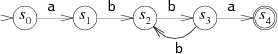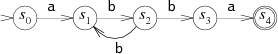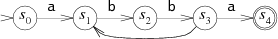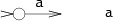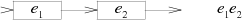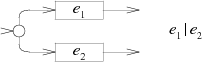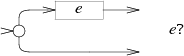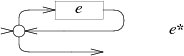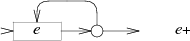# 正则表达式语法

• $x$，$x$是一个简单字符，匹配一个同样的字符
• $xy$，其中$x$和$y$都是正则表达式，匹配一段文本$ab$，其中$x$与$a$匹配，$y$与$b$匹配。
• $x|y$，其中$x$和$y$都是正则表达式，匹配文本$a$，其中$x$与$a$匹配或$y$与$a$匹配。
• $x?$，$x+$，$x*$，分别表示匹配正则表达式$x$最多1一次，至少一次，任意次。

# 自动机